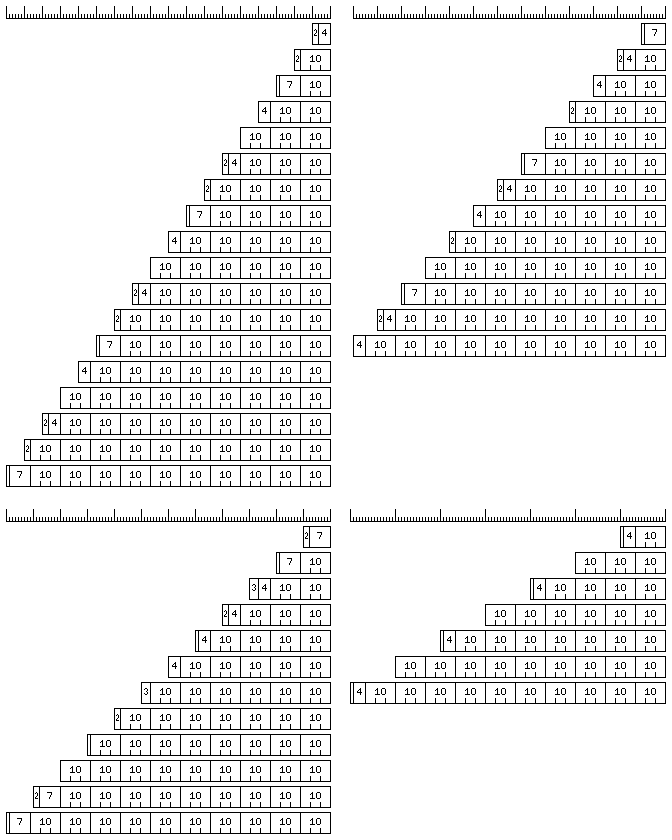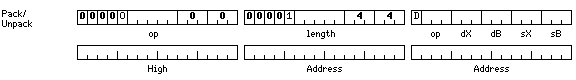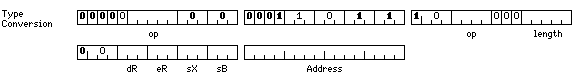[Next] [Up] [Previous]

# Compressed Decimal Numbers

In addition to register packed numbers, simple floating-point numbers, and decimal exponent floating-point numbers, another data type made available by means of this group of instruction prefixes is the compressed decimal data type.

This data type is easier to understand in the case when the word size is either 30 bits or 40 bits and the standard character size of 10 bits is selected, and the format of this type in the simplest case will therefore be explained first.

Versions of the register packed instructions with this prefix are also present, and they follow the same standard pattern applied to the regular packed decimal instructions when the word size is either 30 bits or 40 bits independently of which character size is selected.

In this case, instead of decimal quantities being encoded according to the conventional binary-coded decimal encoding, at the rate of four bits per digit:

```0000 0      0101 5
0001 1      0110 6
0010 2      0111 7
0011 3      1000 8
0100 4      1001 9
```

they are encoded in a manner in which ten bits represent three decimal digits which is known as Chen-Ho encoding.

In this encoding, digits are divided into two classes, normal and high, with the digits from 0 to 7 being normal, and the two digits 8 and 9 being high. The digits themselves are coded as follows:

```Normal                High

000 0    100 4        0 8
001 1    101 5        1 9
010 2    110 6
011 3    111 7
```

and, if a sequence of three digits is represented by either aaa, bbb, and ccc for the three digits if they are normal digits, or A, B, and C for the three digits if they are high digits, the overall format of the ten-bit representation of three digits is coded as follows:

```0aaabbbccc
100Abbbccc
101aaaBccc
110aaabbbC
11100ABccc
11101AbbbC
11110aaaBC
1111100ABC
```

with only the twenty-four combinations of the form 11111nnxxx where the two bits nn are not both zero being unused.

Also note that since only the ten decimal digits are available in this mode, packed decimal quantities in this form are in ten's complement format prior to encoding, as opposed to the modified ten's complement format normally used in which the first digit has sixteen possible values.

In the case of register packed operands for word lengths other than 40 or 60 bits, as much of the word as possible, beginning at the least significant end, is divided up into 10-bit symbols representing three decimal digits. As much of what remains as is possible is then divided into 7-bit symbols representing two decimal digits, coded as follows:

```0aaabbb
100Abbb
101aaaB
11100AB
```

(the code for the last combination being 11100AB instead of 11000AB to permit greater commonality of circuitry between 10-bit and 7-bit encoding and decoding) and then as much of what remains as is possible is divided up into regular four-bit packed decimal digits. If any bits remain, they become a leading digit which will be two bits long, having the values 0, 1, 2, or 3.

If the leading digit is a four-bit packed decimal digit, ten of its possible values begin a positive quantity, and the remaining six values are used to represent negative quantities. In all other cases, half the possible values of the leading digit represent positive quantities, and the other half represent negative quantities.

As a bit of the Program Status Block selects between ten's complement and sign-magnitude notation for decimal numbers, the following table will show the possible meanings assigned to the first digit of a register packed number for both choices in these cases:

```0   00  +0       0   00  +0       0   00  +0       0   00  +0       0   00  +0
1   01  +1       1   01  +1       1   01  +1       1   01  +1       1   99  -0
2   02  +2       2   02  +2       2   02  +2       2   98  -0
3   03  +3       3   03  +3       3   03  +3       3   99  -1
4   04  +4       4   04  +4       4   96  -0
5   05  +5       5   95  -0       5   97  -1
6   06  +6       6   96  -1       6   98  -2
7   07  +7       7   97  -2       7   99  -3
8   08  +8       8   98  -3
9   09  +9       9   99  -4
A   94  -0
B   95  -1
C   96  -2
D   97  -3
E   98  -4
F   99  -5
```

Thus, the overall format makes use of Chen-Ho encoding, but if extra bits are available in the area allocated to a decimal number, then one or more additional digits or partial digits are added in conventional form.

 Note that when sign-magnitude notation rather than ten's complement notation is selected, it may be possible to select IBM's Densely Packed Decimal encoding instead, a bit in the Program Status Block being reserved for such a selection, should this encoding be licensed by implementors. This encoding is not applicable to ten's complement notation. However, while it is possible to modify the Densely Packed Decimal format so that it can be used with decimal numbers in ten's complement notation, the fact that this architecture attempts to use every bit of the area available for a decimal operand, even if that means partial digits are used would still prevent deriving the benefit offered by that format of being able to truncate decimal numbers while they are in compressed form, since truncation points other than decimal digit boundaries are required. However, the Densely Packed Decimal encoding could still confer benefits when decimal numbers are stored on external media or communicated, and furthermore it is expected to form part of forthcoming IEEE standards for numeric representation. As the internal representation of compressed decimal quantities uses all bits of fields whose length is not necessarily suited to quantities consisting of an integral number of digits, it cannot, in any event, be fully conformant to those standards. But the possibility that Densely Packed Decimal encoding may become the prevailing standard, leading to that being used instead of the particular form of Chen-Ho encoding described above, should be considered.

Thus, the numeric ranges for register packed operands under different word lengths in ten's complement operation are shown below (in all cases, the range of negative numbers is decreased by one for sign-magnitude operation):

```Normal:

24                                  -600,000
999,999

48                          -600,000,000,000
999,999,999,999

60                      -600,000,000,000,000
999,999,999,999,999

120  -600,000,000,000,000,000,000,000,000,000
999,999,999,999,999,999,999,999,999,999

32                               -60,000,000
99,999,999

64                    -6,000,000,000,000,000
9,999,999,999,999,999

36                              -600,000,000
999,999,999

72                  -600.000,000,000,000,000
999,999,999,999,999,999

40                            -6,000,000,000
9,999,999,999

80               -60,000,000,000,000,000,000
99,999,999,999,999,999,999

Compressed:

24                                        -6,000,000
9,999,999

48                              -100,000,000,000,000
99,999,999,999,999

60                          -500,000,000,000,000,000
499,999,999,999,999,999

120  -500,000,000,000,000,000,000,000,000,000,000,000
499,999,999,999,999,999,999,999,999,999,999,999

32                                    -2,000,000,000
1,999,999,999

64                        -6,000,000,000,000,000,000
9,999,999,999,999,999,999

36                                   -20,000,000,000
19,999,999,999

72                    -2,000,000,000,000,000,000,000
1,999,999,999,999,999,999,999

40                                  -500,000,000,000
499,999,999,999

80                  -500,000,000,000,000,000,000,000
499,999,999,999,999,999,999,999
```

In the case of the regular packed decimal instructions, the operand lengths specified in the instructions are expressed in characters rather than in digits, and may not lead to a number containing more than 32 decimal digits.

When the string character size is 10 bits, lengths from 1 to 10 characters, leading to precisions from 3 to 30 digits, are possible.

The diagram below:shows how packed decimal operands are organized when compressed decimal operation is in effect for string character sizes of 6, 8, 9 or 15 bits. Note that because 9 and 15 are odd numbers, packed decimal operands in the circumstance of one of those string character sizes, with a length that is an odd number of characters, may begin with a first digit that is either one or three bits in length, as described above.

Note that in the case of a 15 bit string character size, packed decimal operands merely alternate between those composed entirely of 10-bit representations of three digits, and those which begin with one one-bit digit followed by one four-bit digit. The 6 and 8 bit sizes progress through five different starting arrangements, and the 9 bit size progresses through a cycle of ten starting arrangements.

The opcodes for compressed decimal computations are shown below, first those for the compressed decimal versions of the register packed instructions:

```000600 121xxx  RCDC   Register Compressed Decimal Compare
000600 122xxx  RCDME  Register Compressed Decimal Multiply Extensibly
000600 123xxx  RCDDE  Register Compressed Decimal Divide Extensibly
000600 124xxx  RCDA   Register Compressed Decimal Add
000600 125xxx  RCDS   Register Compressed Decimal Subtract
000600 126xxx  RCDM   Register Compressed Decimal Multiply
000600 127xxx  RCDD   Register Compressed Decimal Divide

000600 131xxx  RCDCL  Register Compressed Decimal Compare Long
000600 132xxx  RCDMEL Register Compressed Decimal Multiply Extensibly Long
000600 133xxx  RCDDEL Register Compressed Decimal Divide Extensibly Long
000600 134xxx  RCDAL  Register Compressed Decimal Add Long
000600 135xxx  RCDSL  Register Compressed Decimal Subtract Long
000600 136xxx  RCDML  Register Compressed Decimal Multiply Long
000600 137xxx  RCDDL  Register Compressed Decimal Divide Long
```

and then the form for the compressed decimal versions of the regular packed decimal instructions:and the opcodes:

```000600 010033 00040xx         MESTCD Multiply Extensibly and Store Compressed Decimal
000600 010033 00041xx         CCD    Compare Compressed Decimal
000600 010033 00042xx         MVCD   Move Compressed Decimal
000600 010033 00043xx         DSTRCD Divide and Store Remainder Compressed Decimal
000600 010033 00044xx         ACD    Add Compressed Decimal
000600 010033 00045xx         SCD    Subtract Compressed Decimal
000600 010033 00046xx         MCD    Multiply Compressed Decimal
000600 010033 00047xx         DCD    Divide Compressed Decimal
```

The compressed decimal versions of the pack and unpack instructions have the form:and the opcodes:

```000600 004x44 02xxxx          PCD    Pack to Compressed Decimal
000600 004x44 12xxxx          PHCD   Pack Halfword to Compressed Decimal
000600 004x44 03xxxx          UCD    Unpack from Compressed Decimal
000600 004x44 13xxxx          UHCD   Unpack Halfword from Compressed Decimal
```

The compressed decimal versions of the type conversion operations have the form:and the opcodes:

```000600 011011 1000xx 00xxxx   CVCDB  Convert Compressed Decimal to Byte
000600 011011 1004xx 00xxxx   CVBCD  Convert Byte to Compressed Decimal
000600 011011 1010xx 00xxxx   CVCDH  Convert Compressed Decimal to Halfword
000600 011011 1014xx 00xxxx   CVHCD  Convert Halfword to Compressed Decimal
000600 011011 1020xx 00xxxx   CVCDW  Convert Compressed Decimal to Word
000600 011011 1024xx 00xxxx   CVWCD  Convert Word to Compressed Decimal
000600 011011 1030xx 00xxxx   CVCDL  Convert Compressed Decimal to Long
000600 011011 1034xx 00xxxx   CVLCD  Convert Long to Compressed Decimal
000600 011011 1040xx 00xxxx   CVCDM  Convert Compressed Decimal to Medium
000600 011011 1044xx 00xxxx   CVMCD  Convert Medium to Compressed Decimal
000600 011011 1050xx 00xxxx   CVCDF  Convert Compressed Decimal to Floating
000600 011011 1054xx 00xxxx   CVFCD  Convert Floating to Compressed Decimal
000600 011011 1060xx 00xxxx   CVCDD  Convert Compressed Decimal to Double
000600 011011 1064xx 00xxxx   CVDCD  Convert Double to Compressed Decimal
000600 011011 1070xx 00xxxx   CVCDQ  Convert Compressed Decimal to Quad
000600 011011 1074xx 00xxxx   CVQCD  Convert Quad to Compressed Decimal
```

As well, conversions between compressed decimal and simple floating are provided, by applying the relevant prefix to the instructions above, with these opcodes:

```000400 000600 011011 1010xx 00xxxx  CVCDSFH  Convert Compressed Decimal to Simple Floating Halfword
000400 000600 011011 1014xx 00xxxx  CVSFHCD  Convert Simple Floating Halfword to Compressed Decimal
000400 000600 011011 1020xx 00xxxx  CVCDSF   Convert Compressed Decimal to Simple Floating
000400 000600 011011 1024xx 00xxxx  CVSFCD   Convert Simple Floating to Compressed Decimal
000400 000600 011011 1030xx 00xxxx  CVCDSFL  Convert Compressed Decimal to Simple Floating Long
000400 000600 011011 1034xx 00xxxx  CVSFLCD  Convert Simple Floating Long to Compressed Decimal
```

Note the use of two prefixes. The type extension prefixes, of the form 0 000 0xx xxx 000 000 are treated in this way; other prefixes, of the form 0 000 1xx xxx 000 000 are not compounded, but instead, a series of multiple prefix halfwords simply creates a new prefix chosen from a larger pool, with an independent meaning.

Note that since the length of compressed decimal numbers used with compressed decimal register packed instructions matches that of the register referenced in the instruction, they are not expanded to BCD form in the fixed-point arithmetic registers which are their register operands, and thus mixing ordinary register packed instructions and compressed decimal register packed instructions is not an available method for converting to or from compressed decimal.

This is also why no opcodes are used for compressed decimal load and store instructions, as the ordinary integer load and store instructions perform the same operation. Hence, each time an operand in a register is referenced, just as each time an operand in memory is referenced, it is converted from its encoded form in order for arithmetic to be performed.

An alternative that might be considered, to permit arithmetic to be performed on quantities that occupy the limited amount of space allotted to quantities in compressed decimal form, for use with register packed decimal instructions, would be to simply convert groups of three decimal digits to binary numbers having values from 0 to 999 and occupying 10 bits. One could even apply excess-12 notation to groups of three digits, on the same principle that allows excess-3 notation to facilitate doing arithmetic on decimal quantities in an arithmetic-logic unit primarily designed for binary arithmetic.

While this numeric format would allow addition and subtraction to be performed on compressed decimal quantities, it would lead to considerably more circuitry being required to perform multiplication and division, and they would still be performed more slowly than with conventional binary-coded decimal numbers.

[Next] [Up] [Previous]